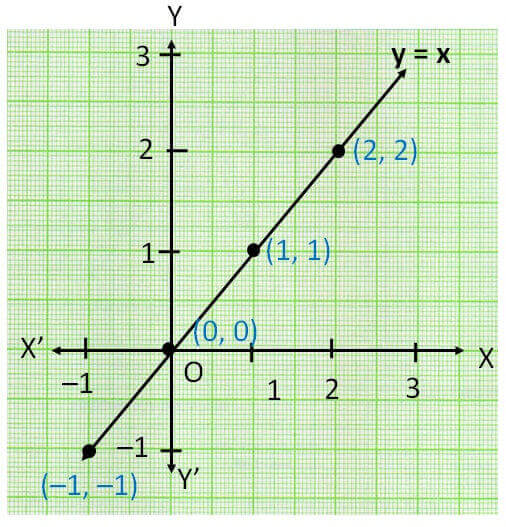Lines parallel x or y axis

Chapter 4 Class 9 Linear Equations in Two Variables
Concept wise

Equation of lines passing through origin are

y = kx

where k is a constant

Let us check an example

y = x

Drawing y = x in graph

Points which satisfy the equation is

 x 0 1 2 –1 y 0 1 2 –1

Plotting points on graphHence, y = x is  line which passes through origin

Get live Maths 1-on-1 Classs - Class 6 to 12